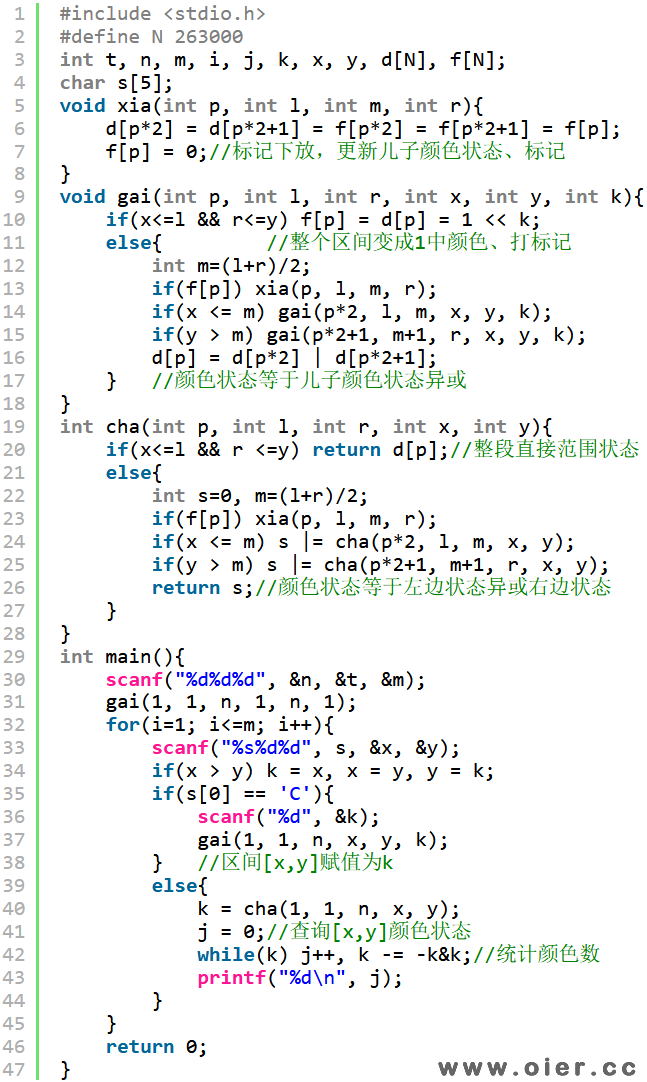POJ2777CountColor
1186+

### Description

Chosen Problem Solving and Program design as an optional course, you are required to solve all kinds of problems. Here, we get a new problem.

There is a very long board with length L centimeter, L is a positive integer, so we can evenly divide the board into L segments, and they are labeled by 1, 2, … L from left to right, each is 1 centimeter long. Now we have to color the board – one segment with only one color. We can do following two operations on the board:

1. “C A B C” Color the board from segment A to segment B with color C.

2. “P A B” Output the number of different colors painted between segment A and segment B (including).

In our daily life, we have very few words to describe a color (red, green, blue, yellow…), so you may assume that the total number of different colors T is very small. To make it simple, we express the names of colors as color 1, color 2, … color T. At the beginning, the board was painted in color 1. Now the rest of problem is left to your.

### Input

First line of input contains L (1 <= L <= 100000), T (1 <= T <= 30) and O (1 <= O <= 100000). Here O denotes the number of operations. Following O lines, each contains “C A B C” or “P A B” (here A, B, C are integers, and A may be larger than B) as an operation defined previously.

Output

Ouput results of the output operation in order, each line contains a number.

### Sample Input

2 2 4
C 1 1 2
P 1 2
C 2 2 2
P 1 2


### Sample Output

2
1


# 程序实现